# Demystifying activation functions in neural network

OK, so what is an activation function? If you don’t know, let me give you a bookish definition.

An activation function is a mathematical function used in a neural network that activates the neurons and introduce non-linearity by transformation of the inputs. They are also known as Transfer Functions.

Now, we all know such definitions, and apply the activation functions in our daily deep learning problems. But, here in this article I will try to answer most of the doubts we have regarding activation functions with very minimal math and lots of intuition.

## Prerequisite

1. You have some understanding on how a deep neural network works, may be a simple feed forward neural network.
2. Basic idea of numerical optimizations like, Gradient Descent.
3. Basic idea on how back propagation works.

## Contents

1. What is the actual purpose of the activation function?
2. Different types of activation functions.

# What is the actual purpose of the activation function?

## Activate Neuron

Let’s start with the phrase that activation functions do activate neurons. So, what is the meaning of activating a neuron. In simple, an activation function controls that if a neuron will give some output or not. For example we can think a step function as an activation function.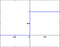sample google image for step function

You can see that, if the value of ‘x’ is greater than zero, then the activation function (step function) gives and output as ‘1’ otherwise ‘0’. Some sort of how does a switch works (ON & OFF). Based on the input value (here ‘x’) the activation function (step function) is either ‘firing’ the neuron (output=1) or ‘not firing’ it (output=0).

Imagine you are designing a classification (binary) model by using the ‘step function’ as your activation function. So it’s quite easy, isn’t it? If you get an output=1 so it belongs to a correct class otherwise it’s not. So, basically a step function is nothing but some sort of ‘max()’ function, to be specific ‘hard max’ function. This ‘hard’ is something where the main drawback arises. If you try to do a multi-class classification problem, you might end up using multiple step functions as well. But, you can see that sometimes more than one step functions are giving you output=1, then how would you arrive at the conclusion of the correct class? Don’t you think if you get the output with some weights (probabilities) associated with it, then life would have been easier? But doing this thing with step function (hard max function) is quite difficult, that’s why we have something called “soft-max” (some sort of weights/ probabilistic approach) activation function! Now you understood why we used “softmax” in a classification task at the last layer (mostly) and why the function is called “soft”max. Don’t you?

## Reduction of compute complexity

For simplicity, consider designing a fully connected feed forward neural network. A hidden layer equation can be written as below.

Here ‘f’ is the activation function we are talking about. Now imagine a situation if there are no activation functions. Then the whole equation would become something like below.

So, the deep neural network we were trying to design that drills down to a simple linear network. Alternatively, without activation functions, we’ve just added a lot more parameters to the model, making it slower to train and make inference from, without any proper benefit.

## Non-Linearity

In the previous section we discussed that, a deep neural network with multiple layers without activation functions are nothing but a linear network. This linear transformation is also not able to model most of the real world data-set. Real world data are always non linear. Considering a classification problem, if classes are always linearly separable then there is no meaning of using DNNs. Activation functions do create non-linear decision surfaces to separate complex non-linearly separable classes.

Let’s consider the below classification data.

This data is not linearly separable. At least by a single straight line we can’t separate the classes. So a single layer single neuron network (logistic regression) can’t solve our problem. Even a multi layer multi neuron network without non-liner activation functions also can’t solve the problem, because it’s a cascaded linear network.

So, if we analyze properly, this particular data can be classified properly with two linear surfaces. something like below.

So we can use two logistic regression model (two linear surfaces) to classify it properly. The same thing and with more generalized version is called a deep neural network with multiple hidden layers and multiple neurons. Multiple linear surfaces are created and the activation function just give those linear surfaces a non-linear shape. Something like below.

# Different types of activation functions

## Sigmoid

We have seen step function, and sigmoid is some sort of smoothed version of step function. below we have the mathematical formula and the curve.

You don’t have to look at the equation, just concentrate at the curve of sigmoid. Isn’t it looking like the step function? although it’s centered around 0.5, so we have our default threshold at 0.5, but we can increase or decrease the threshold as per the problem.

We have used sigmoid in logistic regression, though sigmoid it self is non-linear, but after it is applied to the weight combination of the LR to make a linear weight transformation. This is the reason a single layer single neuron neural network with sigmoid activation mimics nothing but logistic regression. Multiple layers and multiple sigmoid activation creates non-linearity.

Advantages of sigmoid

1. Sigmoid is used as a squashing function which squashes values between 0 and 1 and acts like a normalizer function.
2. The bound of sigmoid is [0, 1]. So we get a proper probabilistic interpretation which helps in deciding outputs. A generalized version of sigmoid is softmax function.

Disadvantages of sigmoid

1. Sigmoid function consists of exponential term, which is computationally expensive.
2. Sigmoid has a problem of vanishing gradient.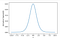Derivative of Sigmoid

The plot above is for the derivative of sigmoid. If you notice that for high and low values of x, the derivative is very low (<<1), so while optimization, due to this very very low gradient value the weights are not updates which in turn causes the vanishing gradient problem.

## Hyperbolic Tangent

We have seen sigmoid function in depth. Hyperbolic tangent function is similar to sigmoid. below we have the mathematical formula and the curve.

If you look at the curve, it’s exactly similar to sigmoid curve, but someone stretched it, so that it is centered around the origin.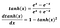Derivative of TanH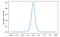Derivative of TanH curve

Advantages of Hyperbolic Tangent

1. TanH is also, used as a squashing function which squashes values between -1 and 1. As it is centered around zero, so it acts as a better implicit normalizer function than sigmoid.
2. If you carefully notice the curve of derivative of TanH, it’s similar like the one we got from derivative of sigmoid, the only difference is that, derivative of TanH has higher values than the derivative of sigmoid. So by using TanH there is a less chance of vanishing gradient than sigmoid.

Disadvantages of Hyperbolic Tangent

1. TanH function consists of exponential term, which is computationally expensive.
2. Still it has a problem of vanishing gradient like sigmoid that we have explained earlier.

## Rectified Linear Unit (ReLU)

The ReLU activation function can be defined as below.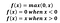ReLU definition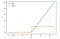ReLU (blue) and derivative of ReLU (yellow)

If you notice the equation and the graphs of ReLU and it’s first derivative, I hope you will find all the answers. If not then let me explain it for you.

ReLU is simply a switching function, if the value of the input is less than zero then the output will be simply zero. On the other hand if the input is greater than zero then the output will be same as the input value.

Now, you might have a good question, that, ReLU seems a linear function then how does it introduce non-linearity? Now I would suggest that you should look at the curve again, at ‘0’ there is a break-point making it non-linear.

Advantages of ReLU

1. ReLU has no complex term, so it’s compute inexpensive.
2. ReLU solves the problem of vanishing gradient. For higher values of ‘x’ the derivative of ReLU is always ‘1’, so there will be no degradation of the error signal while back-propagation.

Disadvantage of ReLU

1. From the gradient curve of the ReLU you can see that if the values of ‘x’ are less than ‘0’ then the gradient is also ‘0’. Due to this certain weights can be “killed off” or become “dead”. This is because the back-propagated error can be cancelled out whenever there is a negative input into a given neuron and therefore the gradient will also fall to zero. This means there is no way for the associated weights to update in the right direction. This problem is called as “dead neuron”.

## Leaky ReLU

Leaky ReLU can be defined as below.

Leaky ReLU is the improved version of ReLU and solves the problem of “dead neuron” by defining an extremely small linear component of x as ou can notice in the curve above.

Leaky ReLU can be further generalized by making the per-determined slope (0.01) as a parameter to the neural network. This version is called Parametric ReLU.

# Conclusion

Likewise, there are many other activation functions are available like ELU, GELU, SELU, MAXOUT etc.

But, the main motivation of this post to make you understand intuitively about how activation function works, and how to get to know about which activation function you need to work on.

Till then Happy Machine Learning :)

## More from sourajit roy chowdhury

Machine learning Engineer